# SQL SERVER – Computed Columns – Index and Performance

This is the last article in the series of the computed columns I have been writing. Here are previous articles.

SQL SERVER – Computed Column – PERSISTED and Storage

This article talks about how computed columns are created and why they take more storage space than before.

SQL SERVER – Computed Column – PERSISTED and Performance

SQL SERVER – Computed Column – PERSISTED and Performance – Part 2

SQL SERVER – Computed Column and Performance – Part 3

SQL SERVER – Computed Column – PERSISTED and Storage – Part 2

This article talks about how creating index on computed column does not grow the row length of table.

SQL SERVER – Computed Columns – Index and Performance

In this article today, we will see how we can get better performance using computed column. Here are few steps which we are going to follow. First, we will create regular table and populate with some data. Once data is populated we will try to query the data. We will get the table scan because there is no table. After this, we will create index and see that we will still get Index Scan because of computation and not Index Seek (which is desirable). These actions will be followed by the creation of the computed column and index on the computed column. We can right away see the improvement of the performance as Index Seek will be applied. Let us understand the same with an example.

```USE [tempdb]
GO
IF EXISTS (SELECT * FROM sys.objects WHERE OBJECT_ID = OBJECT_ID(N'[dbo].[CompCol]') AND TYPE IN (N'U'))
DROP TABLE [dbo].[CompCol]
GO
CREATE TABLE [dbo].[CompCol](
[ID] [int] NOT NULL,
[FirstName] [varchar](100) NULL,
[LastName] [varchar](100) NULL,
[BirthDate] [datetime] NULL,
CONSTRAINT [PK_CompCol] PRIMARY KEY CLUSTERED
([ID] ASC)
)
GO

```

` `

Let us now Insert few rows into the table.

```-- Insert One Hundred Thousand Records
INSERT INTO CompCol (ID,FirstName,LastName, BirthDate)
SELECT TOP 100000 ROW_NUMBER() OVER (ORDER BY a.name) RowID,
'Bob',
CASE WHEN ROW_NUMBER() OVER (ORDER BY a.name)%2 = 1 THEN 'Smith'
ELSE 'Brown' END,
CASE WHEN ROW_NUMBER() OVER (ORDER BY a.name)%2 = 1 THEN DATEADD(yy,-2, '2010-08-13 14:20:24.853')
WHEN ROW_NUMBER() OVER (ORDER BY a.name)%3 = 1 THEN DATEADD(yy,-3, '2010-08-13 14:20:24.853')
WHEN ROW_NUMBER() OVER (ORDER BY a.name)%5 = 1 THEN DATEADD(yy,-5, '2010-08-13 14:20:24.853')
WHEN ROW_NUMBER() OVER (ORDER BY a.name)%7 = 1 THEN DATEADD(yy,-7, '2010-08-13 14:20:24.853')
ELSE GETDATE() END
FROM sys.all_objects a
CROSS JOIN sys.all_objects b
GO

```

Now we will apply a simple SELECT statement on the table.

```-- Select specific year data
SELECT ID, FirstName
FROM CompCol
WHERE MONTH(BirthDate) = 8
GO

```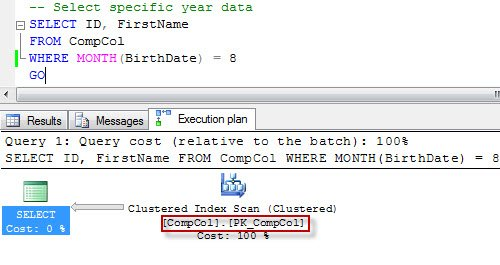From the resultset, it is clear that query is doing index scan. This is natural as there is no index on the table. Let us create an index on the field which is used in the WHERE clause.

```-- Create non clustered index on regular column
CREATE NONCLUSTERED INDEX IX_CompCol_BrithDate
ON dbo.CompCol (BirthDate, FirstName)
GO
```

After creating an index, let us run the original SELECT statement once again. You will notice that Index scan still is there. As we are looking for a specific value in our WHERE condition, Index seek is desirable.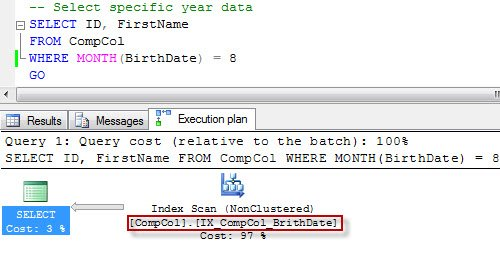Now, let us create the computed column by keeping what we have in WHERE condition. We can right away store the value of the months in a separate column.

```-- Add Computed Column
BirthMonth AS MONTH(BirthDate)
GO
```

However, as mentioned in the earlier articles, computed columns are materialized at the run time. Due the same reason, it cannot stand along improve the performance, and the SELECT statement will give a very similar performance as before.

```-- Select specific year data
SELECT ID, FirstName
FROM CompCol
WHERE BirthMonth = 8
GO
```

Now let us create index on the computed column which we have just created.

```-- Create non clustered index on Computed Column
CREATE NONCLUSTERED INDEX IX_CompCol_BirthMonth
ON dbo.CompCol (BirthMonth, FirstName)
GO
```

After creating index, let us run both the T-SQL Query again. The first one we run is that which had computed column in WHERE condition.

```-- Select specific year data
SELECT ID, FirstName
FROM CompCol
WHERE BirthMonth = 8
GO
```

We will observe that the newly created index is used now and the earlier Index Scan is now converted to Index Seek.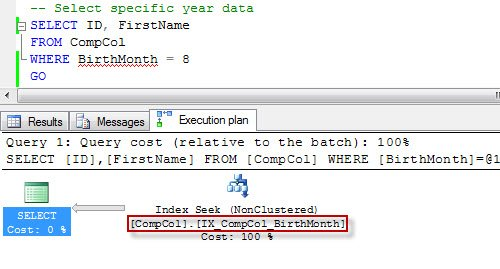Now let us run very first script which had ran where we used MONTH function in WHERE condition.

```-- Compare above query with original Query
SELECT ID, FirstName
FROM CompCol
WHERE MONTH(BirthDate) = 8
GO
```

We can now see that the newly created index is also applied here, and Index Scan is also converted to Index Seek.

We can definitely see that Index Scan has been converted to Index Seek, but does this really improve the performance?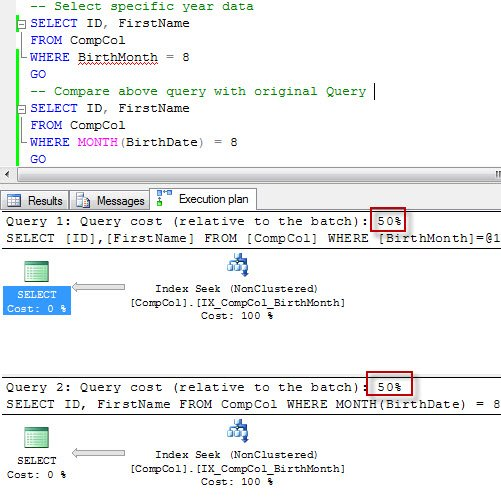We can compare the earlier query which used a different index (forcing index scan) with the newly changed index seek query. To recreate this, we will have to use index hint of the index used earlier.

```-- Compare above query with original Query
SELECT ID, FirstName
FROM CompCol
WHERE MONTH(BirthDate) = 8
GO
-- Compare above query with original Query
-- with hint of original index use
SELECT ID, FirstName
FROM CompCol WITH (INDEX(IX_CompCol_BrithDate))
WHERE MONTH(BirthDate) = 8
GO
```

You can enable the execution plan and clearly observe that after creating a new index on the computed column, the performance has improved.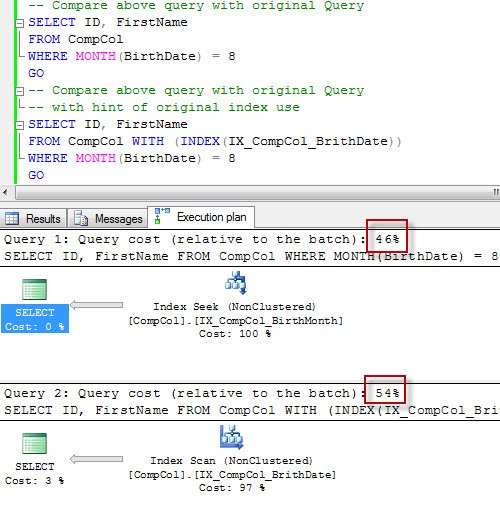You can run following command to clean up.

```-- Clean up Database
DROP TABLE CompCol
GO
```

In summary, this blog post clearly demonstrates that a computed column with index created on it can be useful right away even if you are not using computed column. SQL Server Engine is smart enough to make the right choice.

You can copy the complete code from here:

```USE [tempdb]
GO
IF EXISTS (SELECT * FROM sys.objects WHERE OBJECT_ID = OBJECT_ID(N'[dbo].[CompCol]') AND TYPE IN (N'U'))
DROP TABLE [dbo].[CompCol]
GO
CREATE TABLE [dbo].[CompCol](
[ID] [int] NOT NULL,
[FirstName] [varchar](100) NULL,
[LastName] [varchar](100) NULL,
[BirthDate] [datetime] NULL,
CONSTRAINT [PK_CompCol] PRIMARY KEY CLUSTERED
([ID] ASC)
)
GO
-- Insert One Hundred Thousand Records
INSERT INTO CompCol (ID,FirstName,LastName, BirthDate)
SELECT TOP 100000 ROW_NUMBER() OVER (ORDER BY a.name) RowID,
'Bob',
CASE WHEN ROW_NUMBER() OVER (ORDER BY a.name)%2 = 1 THEN 'Smith'
ELSE 'Brown' END,
CASE WHEN ROW_NUMBER() OVER (ORDER BY a.name)%2 = 1 THEN DATEADD(yy,-2, '2010-08-13 14:20:24.853')
WHEN ROW_NUMBER() OVER (ORDER BY a.name)%3 = 1 THEN DATEADD(yy,-3, '2010-08-13 14:20:24.853')
WHEN ROW_NUMBER() OVER (ORDER BY a.name)%5 = 1 THEN DATEADD(yy,-5, '2010-08-13 14:20:24.853')
WHEN ROW_NUMBER() OVER (ORDER BY a.name)%7 = 1 THEN DATEADD(yy,-7, '2010-08-13 14:20:24.853')
ELSE GETDATE() END
FROM sys.all_objects a
CROSS JOIN sys.all_objects b
GO
-- Select specific year data
SELECT ID, FirstName
FROM CompCol
WHERE MONTH(BirthDate) = 8
GO
-- Create non clustered index on regular column
CREATE NONCLUSTERED INDEX IX_CompCol_BrithDate
ON dbo.CompCol (BirthDate, FirstName)
GO
-- Select specific year data
SELECT ID, FirstName
FROM CompCol
WHERE MONTH(BirthDate) = 8
GO
BirthMonth AS MONTH(BirthDate)
GO
-- Select specific year data
SELECT ID, FirstName
FROM CompCol
WHERE BirthMonth = 8
GO
-- Create non clustered index on Computed Column
CREATE NONCLUSTERED INDEX IX_CompCol_BirthMonth
ON dbo.CompCol (BirthMonth, FirstName)
GO
-- Select specific year data
SELECT ID, FirstName
FROM CompCol
WHERE BirthMonth = 8
GO
-- Compare above query with original Query
SELECT ID, FirstName
FROM CompCol
WHERE MONTH(BirthDate) = 8
GO
-- Compare above query with original Query
-- with hint of original index use
SELECT ID, FirstName
FROM CompCol WITH (INDEX(IX_CompCol_BrithDate))
WHERE MONTH(BirthDate) = 8
GO
-- Clean up Database
DROP TABLE CompCol
GO
```

Reference : Pinal Dave (https://blog.sqlauthority.com)

#### Related Posts

•